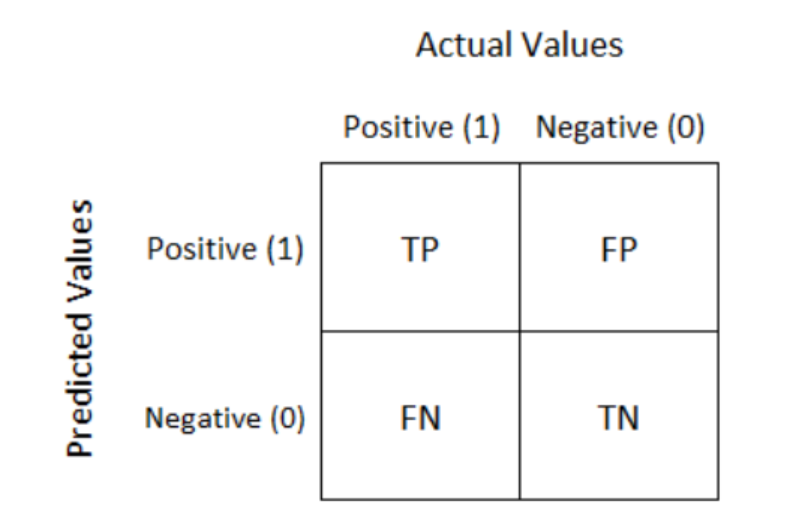# Performance Metrics for Classification Machine Learning ProblemsAccuracy, Precision, Recall, F1 Score, ROC AUC, Log loss. Many learning algorithms have been proposed. It is often valuable to assess the efficacy of an algorithm.

Many learning algorithms have been proposed. It is often valuable to assess the efficacy of an algorithm. In many cases, such assessment is relative, that is, evaluating which of several alternative algorithms is best suited to a specific application.

People even end up creating metrics that suit the application. In this article, we will see some of the most common metrics in a classification setting of a problem.

The most commonly used Performance metrics for classification problem are as follows,

• Accuracy
• Confusion Matrix
• Precision, Recall, and F1 score
• ROC AUC
• Log-loss

# Accuracy

Accuracy is the simple ratio between the number of correctly classified points to the total number of points.

To calculate accuracy, scikit-learn provides a utility function.

``````from sklearn.metrics import accuracy_score

#predicted y values
y_pred = [0, 2, 1, 3]
#actual y values
y_true = [0, 1, 2, 3]
accuracy_score(y_true, y_pred)
0.5``````

Accuracy is simple to calculate but has its own disadvantages.

## Limitations of accuracy

• If the data set is highly imbalanced, and the model classifies all the data points as the majority class data points, the accuracy will be high. This makes accuracy not a reliable performance metric for imbalanced data.
• From accuracy, the probability of the predictions of the model can be derived. So from accuracy, we can not measure how good the predictions of the model are.

# Confusion Matrix

Confusion Matrix is a summary of predicted results in specific table layout that allows visualization of the performance measure of the machine learning model for a binary classification problem (2 classes) or multi-class classification problem (more than 2 classes)Confusion matrix of a binary classification

• TP means True Positive. It can be interpreted as the model predicted positive class and it is True.
• FP means False Positive. It can be interpreted as the model predicted positive class but it is False.
• FN means False Negative. It can be interpreted as the model predicted negative class but it is False.
• TN means True Negative. It can be interpreted as the model predicted negative class and it is True.

For a sensible model, the principal diagonal element values will be high and the off-diagonal element values will be below i.e., TP, TN will be high.

To get an appropriate example in a real-world problem, consider a diagnostic test that seeks to determine whether a person has a certain disease. A false positive in this case occurs when the person tests positive but does not actually have the disease. A false negative, on the other hand, occurs when the person tests negative, suggesting they are healthy when they actually do have the disease.

For a multi-class classification problem, with ‘c’ class labels, the confusion matrix will be a (c*c) matrix.

To calculate confusion matrix, sklearn provides a utility function

## Understanding Performance metrics for Machine Learning Algorithms

Understanding Performance metrics for Machine Learning Algorithms Performance metrics explained — How do they work and when to use which?

## Beginners Guide to Machine Learning on GCP

This blog covers basic knowledge needed to get started ML journey on GCP. Machine Learning is a way to use some set of algorithms to derive predictive analytics from data.

## Most popular Data Science and Machine Learning courses — July 2020

Most popular Data Science and Machine Learning courses — August 2020. This list was last updated in August 2020 — and will be updated regularly so as to keep it relevant

## How To Build A Data Science Career In 2021

In Conversation With Dr Suman Sanyal, NIIT University,he shares his insights on how universities can contribute to this highly promising sector and what aspirants can do to build a successful data science career.

## Learn Data Science Today - Data Science Tutorial for Beginners 2020!

How and why to start Learning to be a data scientist in 2020! This Data Science Course will give you a Step by Step idea about the Data Science Career, Data science Hands-On Projects, roles & salary offered to a Data Scientist!